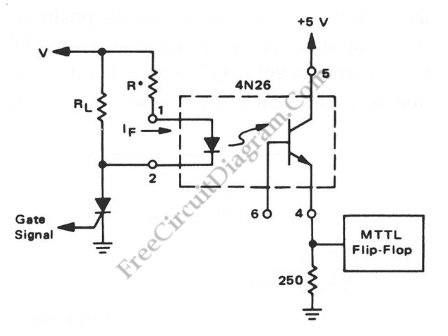# Interfacing High Power Load to Logic Level with OptocouplerInterfacing high power load can be done in many ways, such as using voltage divider resistors, transformer, or optocoupler.  In the circuit shown below , an optocoupler method is presented. This optocoupler serve the logic level translation with flexible input, accepting wide range of voltage level, which is suitable for many high power load/devices. To couple a high-voltage load to a low-voltage logic input, this optocoupler basically convert the signal from the load (input) into light internally, and detected by phototransistor inside. The flexibility of the circuit is made possible by changing the limiter resistor (R*) according to the operating voltage of the load, which is determined by following equation: R=V/0.05*A. To work with TTL loogics, the output current should be greater than 10mA to make sure the output voltage pass the flip-flop’s logic-one level. If dealing only with CMOS logics, then we can increase the 250R resistor to lower the power consumption. Here is the schematic diagram of  the circuit: